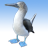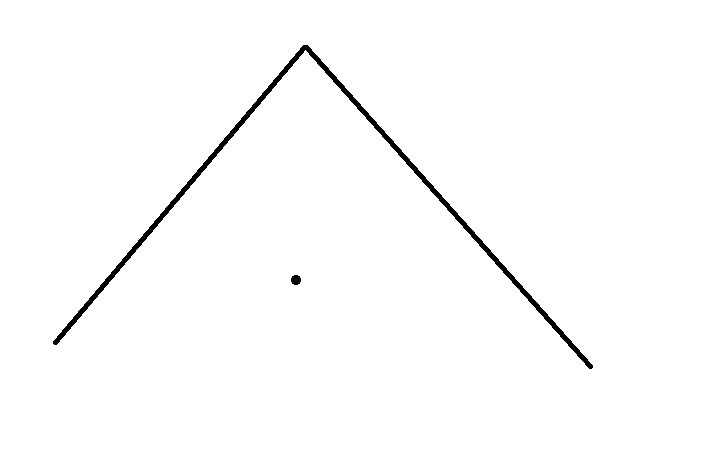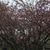# How to find the midpoint of a polyline?

7471
9
04-26-2011 07:52 AMby
New Contributor
Can anyone share with me the easiest way to calculate the midpoint of a polyline on a graphics layer?

TIA
Tags (2)
9 RepliesbyEsri Regular Contributor
`polyline.Extent.GetCenter();`New Contributor III

I think you will not get a midpoint if the line is irregular.New Contributor III

Actually the truecentroid property will give you a gravity center of an irregular polyline but if you want to reach the middle point of a feature you have to do something else.

The picture above is wrong. The centroid property will give you the nearest point from centroid (actually, within the polyline).

Sincerely

Ezequias.New Contributor III

You can do it in Arcpy but I did not find any reference in Javascript.

// Arcpy

Midpoint = geometry.positionAlongLine(0.50,True).firstPoint
... print Midpoint.X
... print Midpoint.YbyEsri Regular Contributor

I think this will be the runtime equivalent method then: GeometryEngine.CreatePointAlong MethodNew Contributor III

Nice to know. Unfortunately I am in a Javascript challengeNew Contributor II

Try the code below. The get_length is another function that just uses the geometryEngine length function. This works for a single part polyline.

function midPointPolyline(line) {
var path = line.paths;
var seglen = get_length(line, "feet");
var midLen = seglen / 2;
var currentDistance = 0;
var beforeIndex = 0;
var startPoint = line.getPoint(0, 0);
for (i = 1; i < path.length - 1; i++) {
var nextPoint = line.getPoint(0, i);
var d = geometryEngine.distance(startPoint, nextPoint, "feet");
if (currentDistance + d < midLen) {
currentDistance += d;
startPoint = nextPoint;
} else {
beforeIndex = (i === 1) ? 0 : i;
break;
}
}
startPoint = line.getPoint(0, beforeIndex);
var endPoint = line.getPoint(0, beforeIndex + 1);
var x = (startPoint.x + endPoint.x) / 2;
var y = (startPoint.y + endPoint.y) / 2;
var midpt = new esri.geometry.Point({ x, y, spatialReference: { wkid: line.spatialReference.wkid } });
return midpnt;
}New Contributor III

(Deleting double post)New Contributor III

Hi @ScottDavis3.  With your help, I was able to get a working solution.  Thank you!  Seems this would be built into the ArcGIS API for JavaScript?!

I have made a couple of changes to Scott's code.  Most notably, I had to change get_length() to geodesicLength() in the geometryEngine class because I couldn't find get_length().

The code below utilizes the ArcGIS for JavaScript 3.x version.

``````function getPolylineMidPoint(polyline) {
const path = polyline.paths;
const length = GeometryEngine.geodesicLength(polyline, "feet"); // esri.geometry.geometryEngine
const middleLength = length / 2;
var currentDistance = 0;
var beforeIndex = 0;
var startPoint = polyline.getPoint(0, 0);
for (let i = 1; i < path.length - 1; i++) {
var nextPoint = polyline.getPoint(0, i);
var d = GeometryEngine.distance(startPoint, nextPoint, "feet");
if (currentDistance + d < middleLength) {
currentDistance += d;
startPoint = nextPoint;
} else {
beforeIndex = (i === 1) ? 0 : i;
break;
}
}
startPoint = polyline.getPoint(0, beforeIndex);
const endPoint = polyline.getPoint(0, beforeIndex + 1);
const x = (startPoint.x + endPoint.x) / 2;
const y = (startPoint.y + endPoint.y) / 2;
const midPoint = new Point({ x, y, spatialReference: { wkid: polyline.spatialReference.wkid } }); // esri.geometry.Point
return midPoint;
};

function doTest () {
const query = new Query(); // esri.tasks.Query
query.where = "Type='Tunnel'";
self._someLayer.queryFeatures(query,
(fs) => {
const features = fs.features;
const midPoint = getPolylineMidPoint(features.geometry); // utilizing the midpoint function
const location = webMercatorUtils.webMercatorToGeographic(midPoint); // esri.geometry.webMercatorUtils
const symbol = new SimpleMarkerSymbol( // "esri.symbols.SimpleMarkerSymbol"
SimpleMarkerSymbol.STYLE_CIRCLE,
15,
new SimpleLineSymbol( // "esri.symbols.SimpleLineSymbol"
SimpleLineSymbol.STYLE_SOLID,
new Color([0, 0, 255, 0.5]),
8
),
new Color([0, 0, 255]) // esri.Color
);
const graphic = new Graphic(location, symbol); // esri.Graphic